• No products in the cart.

# 203.4.4 What is a Best Model?

### What is a best model? How to build?

In previous section, we studied about ROC and AUC

• A model with maximum accuracy /least error
• A model that uses maximum information available in the given data
• A model that has minimum squared error
• A model that captures all the hidden patterns in the data
• A model that produces the best perdition results

## Model Selection

• How to build/choose a best model?
• Error on the training data is not a good meter of performance on future data
• How to select the best model out of the set of available models ?
• Are there any methods/metrics to choose best model?
• What is training error? What is testing error? What is hold out sample error?

### LAB: The Most Accurate Model

• Data: Fiberbits/Fiberbits.csv
• Build a decision tree to predict active_user
• What is the accuracy of your model?
• Grow the tree as much as you can and achieve 95% accuracy.

### Solution

• Model-1
library(rpart)
library(rpart.plot)
## Warning: package 'rpart.plot' was built under R version 3.1.3
Fiber_bits_tree1<-rpart(active_cust~., method="class", control=rpart.control(minsplit=30, cp=0.01), data=Fiberbits)
prp(Fiber_bits_tree1)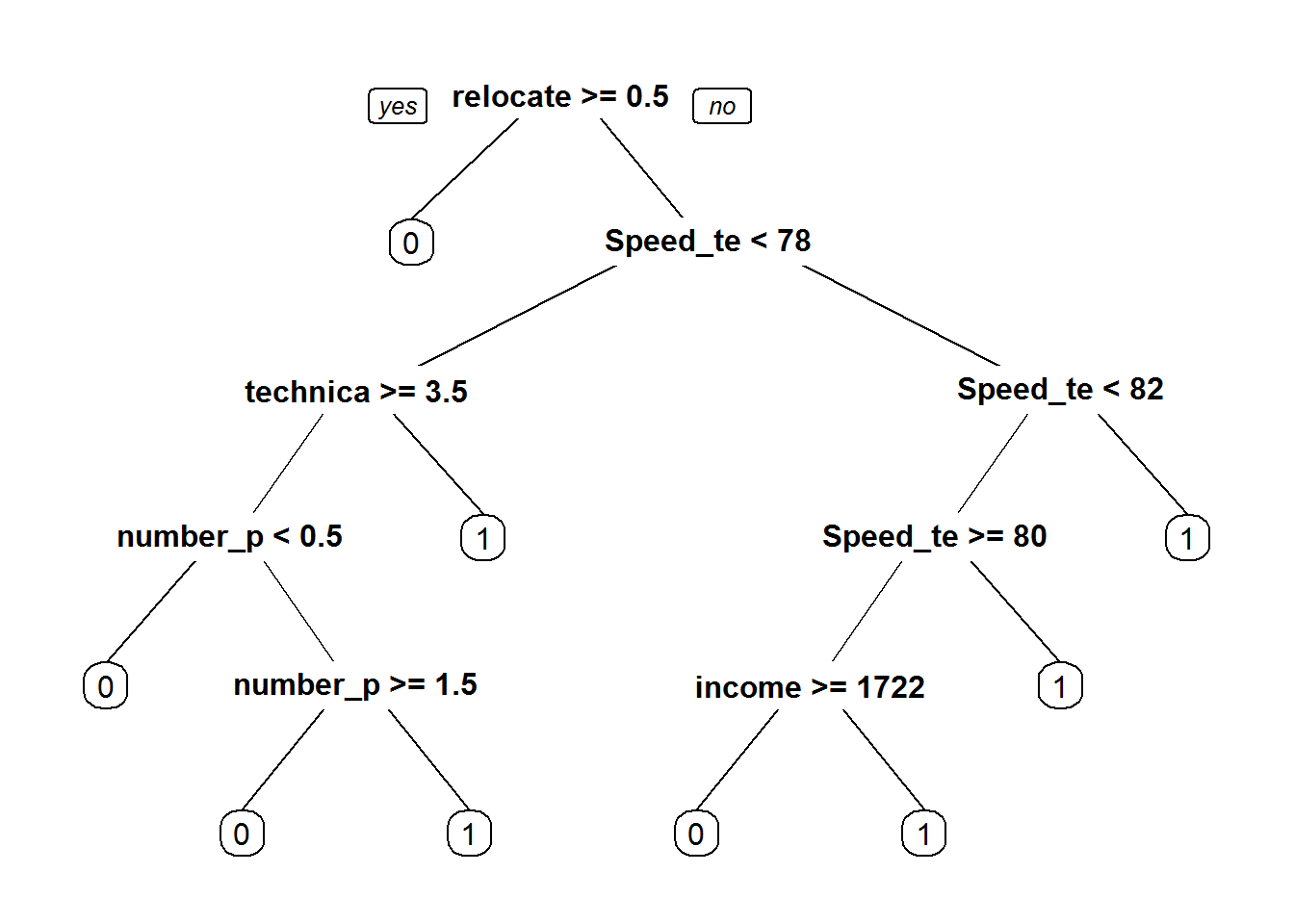Fbits_pred1<-predict(Fiber_bits_tree1, type="class")
conf_matrix1<-table(Fbits_pred1,Fiberbits$active_cust) accuracy1<-(conf_matrix1[1,1]+conf_matrix1[2,2])/(sum(conf_matrix1)) accuracy1 ##  0.84629 • Model-2 Fiber_bits_tree2<-rpart(active_cust~., method="class", control=rpart.control(minsplit=5, cp=0.000001), data=Fiberbits) Fbits_pred2<-predict(Fiber_bits_tree2, type="class") conf_matrix2<-table(Fbits_pred2,Fiberbits$active_cust)
accuracy2<-(conf_matrix2[1,1]+conf_matrix2[2,2])/(sum(conf_matrix2))
accuracy2
##  0.95063

### Different Type of Datasets and Errors

#### The Training Error

• The accuracy of our best model is 95%. Is the 5% error model really good?
• The error on the training data is known as training error.
• A low error rate on training data may not always mean the model is good.
• What really matters is how the model is going to perform on unknown data or test data.
• We need to find out a way to get an idea on error rate of test data.
• We may have to keep aside a part of the data and use it for validation.
• There are two types of datasets and two types of errors

#### Two Types of Datasets

• There are two types of datasets
• Training set: This is used in model building. The input data
• Test set: The unknown dataset. This dataset is gives the accuracy of the final model
• We may not have access to these two datasets for all machine learning problems. In some cases, we can take 90% of the available data and use it as training data and rest 10% can be treated as validation data
• Validation set: This dataset kept aside for model validation and selection. This is a temporary subsite to test dataset. It is not third type of data
• We create the validation data with the hope that the error rate on validation data will give us some basic idea on the test error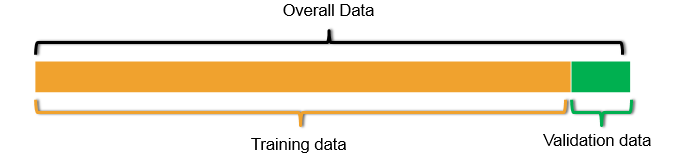#### Types of Errors

• The training error
• The error on training dataset
• In-time error
• Error on the known data
• Can be reduced while building the model
• The test error
• The error that matters
• Out-of-time error
• The error on unknown/new dataset.

“A good model will have both training and test error very near to each other and close to zero”

### The Problem of Over Fitting

• In search of the best model on the given data we add many predictors, polynomial terms, Interaction terms, variable transformations, derived variables, indicator/dummy variables etc.,
• Most of the times we succeed in reducing the error. What error is this?
• So by complicating the model we fit the best model for the training data.
• Sometimes the error on the training data can reduce to near zero
• But the same best model on training data fails miserably on test data.
• Imagine building multiple models with small changes in training data. The resultant set of models will have huge variance in their parameter estimates.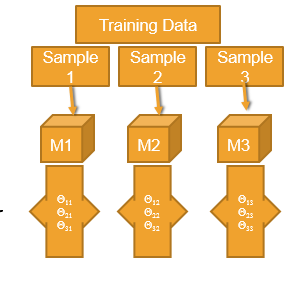• The model is made really complicated, that it is very sensitive to minimal changes
• By complicating the model the variance of the parameters estimates inflates
• Model tries to fit the irrelevant characteristics in the data
• Over fitting
• The model is super good on training data but not so good on test data
• We fit the model for the noise in the data
• Less training error, high testing error
• The model is over complicated with too many predictors
• Model need to be simplified
• A model with lot of variance

### LAB: Model with huge Variance

• Data: Fiberbits/Fiberbits.csv
• Take initial 90% of the data. Consider it as training data. Keep the final 10% of the records for validation.
• Build the best model(5% error) model on training data.
• Use the validation data to verify the error rate. Is the error rate on the training data and validation data same?

### Solution

fiber_bits_train<-Fiberbits[1:90000,]
fiber_bits_validation<-Fiberbits[90001:100000,]

Model on training data

Fiber_bits_tree3<-rpart(active_cust~., method="class", control=rpart.control(minsplit=5, cp=0.000001), data=fiber_bits_train)
Fbits_pred3<-predict(Fiber_bits_tree3, type="class")
conf_matrix3<-table(Fbits_pred3,fiber_bits_train$active_cust) accuracy3<-(conf_matrix3[1,1]+conf_matrix3[2,2])/(sum(conf_matrix3)) accuracy3 ##  0.9524889 Validation Accuracy fiber_bits_validation$pred <- predict(Fiber_bits_tree3, fiber_bits_validation,type="class")

conf_matrix_val<-table(fiber_bits_validation$pred,fiber_bits_validation$active_cust)
accuracy_val<-(conf_matrix_val[1,1]+conf_matrix_val[2,2])/(sum(conf_matrix_val))
accuracy_val
##  0.7116

Error rate on validation data is more than the training data error.

### The Problem of Under-fitting

• Simple models are better. Its true but is that always true? May not be always true.
• We might have given it up too early. Did we really capture all the information?
• Did we do enough research and future reengineering to fit the best model? Is it the best model that can be fit on this data?
• By being over cautious about variance in the parameters, we might miss out on some patterns in the data.
• Model need to be complicated enough to capture all the information present.
• If the training error itself is high, how can we be so sure about the model performance on unknown data?
• Most of the accuracy and error measuring statistics give us a clear idea on training error, this is one advantage of under fitting, we can identify it confidently.
• Under fitting
• A model that is too simple
• A mode with a scope for improvement
• A model with lot of bias

### LAB: Model with huge Bias

• Lets simplify the model.
• Take the high variance model and prune it.
• Make it as simple as possible.
• Find the training error and validation error.

### Solution

• Simple Model
Fiber_bits_tree4<-rpart(active_cust~., method="class", control=rpart.control(minsplit=30, cp=0.25), data=fiber_bits_train)
prp(Fiber_bits_tree4)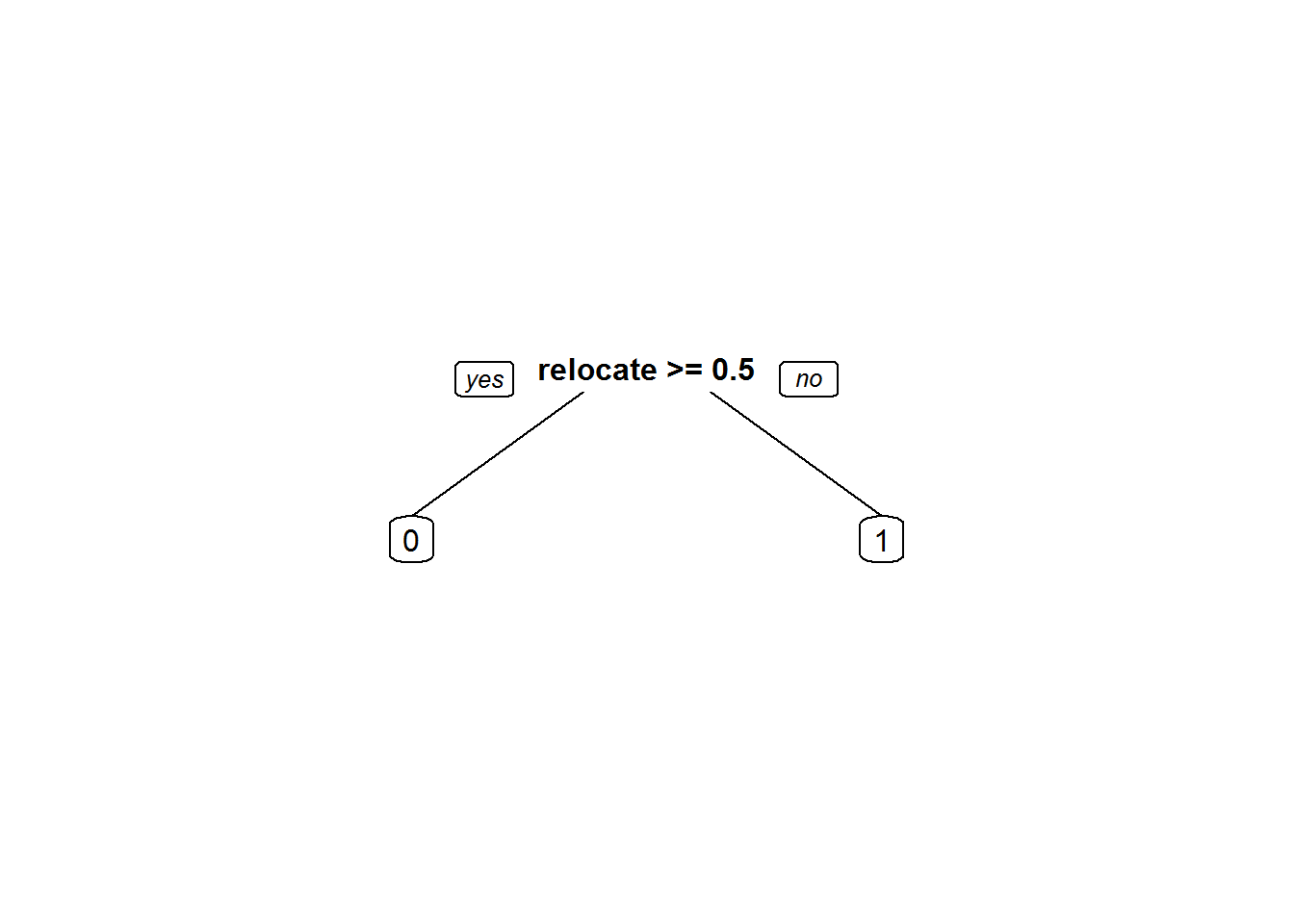Fbits_pred4<-predict(Fiber_bits_tree4, type="class")
conf_matrix4<-table(Fbits_pred4,fiber_bits_train$active_cust) conf_matrix4 ## ## Fbits_pred4 0 1 ## 0 11209 921 ## 1 25004 52866 accuracy4<-(conf_matrix4[1,1]+conf_matrix4[2,2])/(sum(conf_matrix4)) accuracy4 ##  0.7119444 • Validation accuracy fiber_bits_validation$pred1 <- predict(Fiber_bits_tree4, fiber_bits_validation,type="class")

conf_matrix_val1<-table(fiber_bits_validation$pred1,fiber_bits_validation$active_cust)
accuracy_val1<-(conf_matrix_val1[1,1]+conf_matrix_val1[2,2])/(sum(conf_matrix_val1))
accuracy_val1
##  0.4224

### Model Bias and Variance

• Over fitting
• Low Bias with High Variance
• Low training error – ‘Low Bias’
• High testing error
• Unstable model – ‘High Variance’
• The coefficients of the model change with small changes in the data
• Under fitting
• High Bias with low Variance
• High training error – ‘high Bias’
• testing error almost equal to training error
• Stable model – ‘Low Variance’
• The coefficients of the model doesn’t change with small changes in the data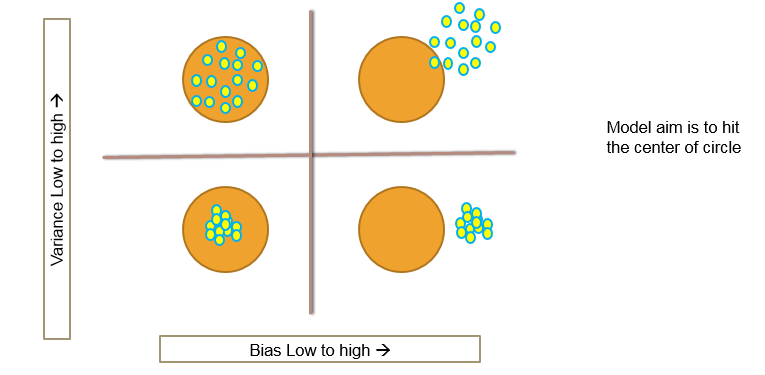### The Bias-Variance Decomposition

$Y = f(X)+\epsilon$ $Var(\epsilon) = \sigma^2$ $Squared Error = E[(Y -\hat{f}(x_0))^2 | X = x_0 ]$ $= \sigma^2 + [E\hat{f}(x_0)-f(x_0)]^2 + E[\hat{f}(x_0)-E\hat{f}(x_0)]^2$ $= \sigma^2 + (Bias)^2(\hat{f}(x_0))+Var(\hat{f}(x_0 ))$

Overall Model Squared Error = Irreducible Error + $Bias^2$ + Variance

### Bias-Variance Decomposition

• Overall Model Squared Error = Irreducible Error + $Bias^2$ + Variance
• Overall error is made by bias and variance together
• High bias low variance, Low bias and high variance, both are bad for the overall accuracy of the model
• A good model need to have low bias and low variance or at least an optimal where both of them are jointly low
• How to choose such optimal model. How to choose that optimal model complexity

### Choosing optimal model-Bias Variance Tradeoff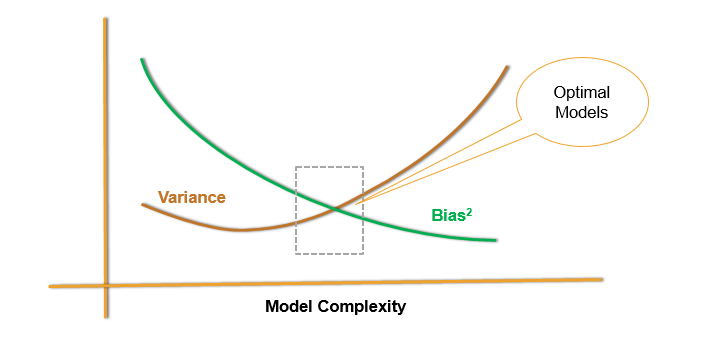### Test and Training Error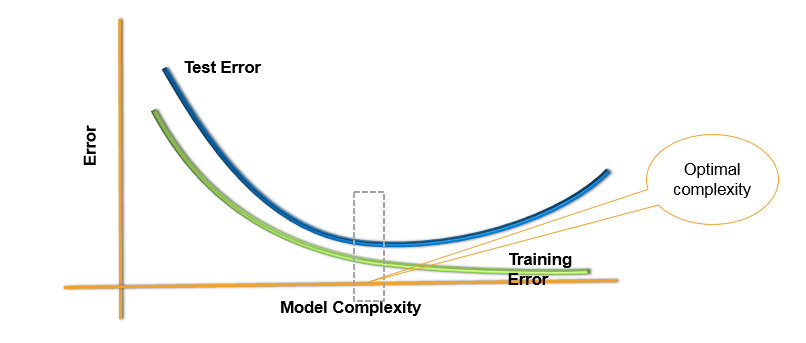### Choosing Optimal Model

• Unfortunately
• There is no scientific method of choosing optimal model complexity that gives minimum test error.
• Training error is not a good estimate of the test error.
• There is always bias-variance tradeoff in choosing the appropriate complexity of the model.
• We can use cross validation methods, boot strapping and bagging to choose the optimal and consistent model

### Holdout Data Cross Validation

• The best solution is out of time validation. Or the testing error should be given high priority over the training error.
• A model that is performing good on training data and equally good on testing is preferred.
• We may not have the test data always. How do we estimate test error?
• We take the part of the data as training and keep aside some potion for validation. May be 80%-20% or 90%-10%
• Data splitting is a very basic intuitive method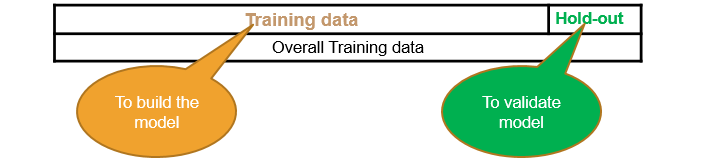### LAB: Holdout Data Cross Validation

• Data: Fiberbits/Fiberbits.csv
• Take a random sample with 80% data as training sample
• Use rest 20% as holdout sample.
• Build a model on 80% of the data. Try to validate it on holdout sample.
• Try to increase or reduce the complexity and choose the best model that performs well on training data as well as holdout data

### Solution

• Caret is a good package for cross validation
library(caret)
sampleseed <- createDataPartition(Fiberbits$active_cust, p=0.80, list=FALSE) train_new <- Fiberbits[sampleseed,] hold_out <- Fiberbits[-sampleseed,] • Model1 library(rpart) Fiber_bits_tree5<-rpart(active_cust~., method="class", control=rpart.control(minsplit=5, cp=0.000001), data=train_new) Fbits_pred5<-predict(Fiber_bits_tree5, type="class") • Accuracy on Training Data conf_matrix5<-table(Fbits_pred5,train_new$active_cust)
conf_matrix5
##
## Fbits_pred5     0     1
##           0 31482  1689
##           1  2230 44599
accuracy5<-(conf_matrix5[1,1]+conf_matrix5[2,2])/(sum(conf_matrix5))
accuracy5
##  0.9510125
• Model1 Validation accuracy
hold_out$pred <- predict(Fiber_bits_tree5, hold_out, type="class") conf_matrix_val<-table(hold_out$pred,hold_out$active_cust) conf_matrix_val ## ## 0 1 ## 0 7003 1333 ## 1 1426 10238 accuracy_val<-(conf_matrix_val[1,1]+conf_matrix_val[2,2])/(sum(conf_matrix_val)) accuracy_val ##  0.86205 • Model2 Fiber_bits_tree5<-rpart(active_cust~., method="class", control=rpart.control(minsplit=30, cp=0.05), data=train_new) Fbits_pred5<-predict(Fiber_bits_tree5, type="class") conf_matrix5<-table(Fbits_pred5,train_new$active_cust)
• Accuracy on Training Data
accuracy5<-(conf_matrix5[1,1]+conf_matrix5[2,2])/(sum(conf_matrix5))
accuracy5
##  0.7882375
• Model2 Validation accuracy
hold_out$pred <- predict(Fiber_bits_tree5, hold_out,type="class") conf_matrix_val<-table(hold_out$pred,hold_out$active_cust) accuracy_val<-(conf_matrix_val[1,1]+conf_matrix_val[2,2])/(sum(conf_matrix_val)) accuracy_val ##  0.79225 • Model3 Fiber_bits_tree5<-rpart(active_cust~., method="class", control=rpart.control(minsplit=30, cp=0.001), data=train_new) Fbits_pred5<-predict(Fiber_bits_tree5, type="class") conf_matrix5<-table(Fbits_pred5,train_new$active_cust)
• Accuracy on Training Data
accuracy5<-(conf_matrix5[1,1]+conf_matrix5[2,2])/(sum(conf_matrix5))
accuracy5
##  0.8673
• Model3 Validation accuracy
hold_out$pred <- predict(Fiber_bits_tree5, hold_out,type="class") conf_matrix_val<-table(hold_out$pred,hold_out$active_cust) accuracy_val<-(conf_matrix_val[1,1]+conf_matrix_val[2,2])/(sum(conf_matrix_val)) accuracy_val ##  0.8661 ### Ten-fold Cross – Validation • Divide the data into 10 parts(randomly) • Use 9 parts as training data(90%) and the tenth part as holdout data(10%) • We can repeat this process 10 times • Build 10 models, find average error on 10 holdout samples. This gives us an idea on testing error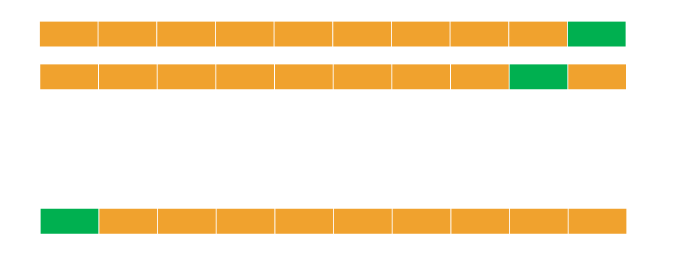### K-fold Cross Validation • A generalization of cross validation. • Divide the whole dataset into k equal parts • Use kth part of the data as the holdout sample, use remaining k-1 parts of the data as training data • Repeat this K times, build K models. The average error on holdout sample gives us an idea on the testing error • Which model to choose? • Choose the model with least error and least complexity • Or the model with less than average error and simple (less parameters) • Finally use complete data and build a model with the chosen number of parameters • Note: Its better to choose K between 5 to 10. Which gives 80% to 90% training data and rest 20% to 10% is holdout data ### LAB – K-fold Cross Validation • Build a tree model on the fiber bits data. • Try to build the best model by making all the possible adjustments to the parameters. • What is the accuracy of the above model? • Perform 10 -fold cross validation. What is the final accuracy? • Perform 20 -fold cross validation. What is the final accuracy? • What can be the expected accuracy on the unknown dataset? ### Solution • Model on complete training data Fiber_bits_tree3<-rpart(active_cust~., method="class", control=rpart.control(minsplit=10, cp=0.000001), data=Fiberbits) Fbits_pred3<-predict(Fiber_bits_tree3, type="class") conf_matrix3<-table(Fbits_pred3,Fiberbits$active_cust)
conf_matrix3
##
## Fbits_pred3     0     1
##           0 38154  2849
##           1  3987 55010
• Accuracy on Traing Data
accuracy3<-(conf_matrix3[1,1]+conf_matrix3[2,2])/(sum(conf_matrix3))
accuracy3
##  0.93164
• k-fold Cross Validation building
• K=10
library(caret)
train_dat <- trainControl(method="cv", number=10)

Need to convert the dependent variable to factor before fitting the model

Fiberbits$active_cust<-as.factor(Fiberbits$active_cust)
• Building the models on K-fold samples
library(e1071)
## Warning: package 'e1071' was built under R version 3.1.3
K_fold_tree<-train(active_cust~., method="rpart", trControl=train_dat, control=rpart.control(minsplit=10, cp=0.000001),  data=Fiberbits)
K_fold_tree$finalModel ## n= 100000 ## ## node), split, n, loss, yval, (yprob) ## * denotes terminal node ## ## 1) root 100000 42141 1 (0.42141000 0.57859000) ## 2) relocated>=0.5 12348 954 0 (0.92274052 0.07725948) * ## 3) relocated< 0.5 87652 30747 1 (0.35078492 0.64921508) ## 6) Speed_test_result< 78.5 27517 10303 0 (0.62557692 0.37442308) * ## 7) Speed_test_result>=78.5 60135 13533 1 (0.22504365 0.77495635) * prp(K_fold_tree$finalModel)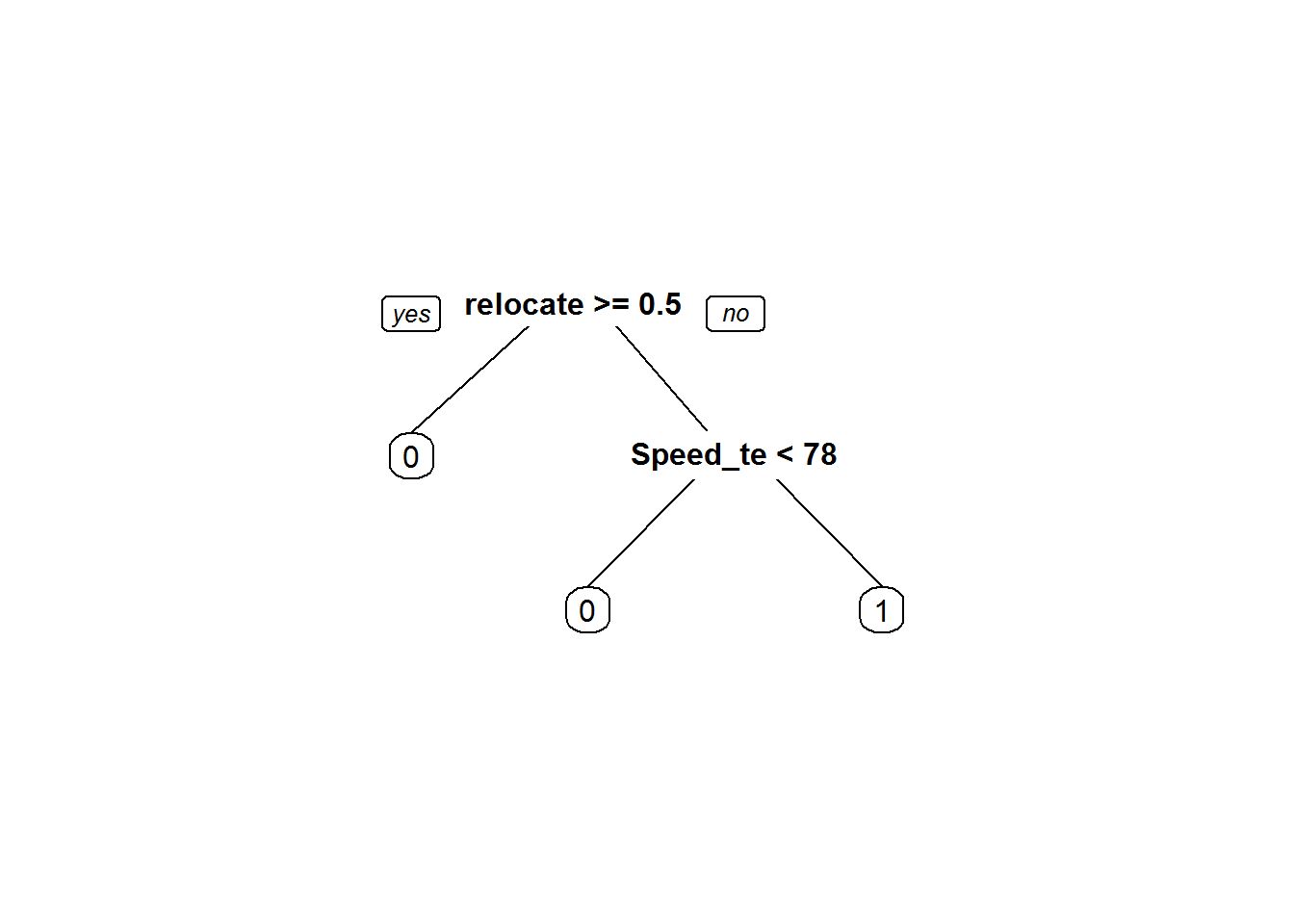Kfold_pred<-predict(K_fold_tree)
conf_matrix6<-confusionMatrix(Kfold_pred,Fiberbits$active_cust) conf_matrix6 ## Confusion Matrix and Statistics ## ## Reference ## Prediction 0 1 ## 0 28608 11257 ## 1 13533 46602 ## ## Accuracy : 0.7521 ## 95% CI : (0.7494, 0.7548) ## No Information Rate : 0.5786 ## P-Value [Acc > NIR] : < 2.2e-16 ## ## Kappa : 0.4879 ## Mcnemar's Test P-Value : < 2.2e-16 ## ## Sensitivity : 0.6789 ## Specificity : 0.8054 ## Pos Pred Value : 0.7176 ## Neg Pred Value : 0.7750 ## Prevalence : 0.4214 ## Detection Rate : 0.2861 ## Detection Prevalence : 0.3987 ## Balanced Accuracy : 0.7422 ## ## 'Positive' Class : 0 ##  • K=20 library(caret) train_dat <- trainControl(method="cv", number=20) Need to convert the dependent variable to factor before fitting the model Fiberbits$active_cust<-as.factor(Fiberbits$active_cust) Building the models on K-fold samples library(e1071) K_fold_tree_1<-train(active_cust~., method="rpart", trControl=train_dat, control=rpart.control(minsplit=10, cp=0.000001), data=Fiberbits) K_fold_tree_1$finalModel
## n= 100000
##
## node), split, n, loss, yval, (yprob)
##       * denotes terminal node
##
## 1) root 100000 42141 1 (0.42141000 0.57859000)
##   2) relocated>=0.5 12348   954 0 (0.92274052 0.07725948) *
##   3) relocated< 0.5 87652 30747 1 (0.35078492 0.64921508)
##     6) Speed_test_result< 78.5 27517 10303 0 (0.62557692 0.37442308) *
##     7) Speed_test_result>=78.5 60135 13533 1 (0.22504365 0.77495635) *
prp(K_fold_tree_1$finalModel)Kfold_pred<-predict(K_fold_tree_1) Caret package has confusion matrix function conf_matrix6_1<-confusionMatrix(Kfold_pred,Fiberbits$active_cust)
conf_matrix6_1
## Confusion Matrix and Statistics
##
##           Reference
## Prediction     0     1
##          0 28608 11257
##          1 13533 46602
##
##                Accuracy : 0.7521
##                  95% CI : (0.7494, 0.7548)
##     No Information Rate : 0.5786
##     P-Value [Acc > NIR] : < 2.2e-16
##
##                   Kappa : 0.4879
##  Mcnemar's Test P-Value : < 2.2e-16
##
##             Sensitivity : 0.6789
##             Specificity : 0.8054
##          Pos Pred Value : 0.7176
##          Neg Pred Value : 0.7750
##              Prevalence : 0.4214
##          Detection Rate : 0.2861
##    Detection Prevalence : 0.3987
##       Balanced Accuracy : 0.7422
##
##        'Positive' Class : 0
## 

## Bootstrap Cross Validation

### Bootstrap Methods

• Boot strapping is a powerful tool to get an idea on accuracy of the model and the test error
• Can estimate the likely future performance of a given modeling procedure, on new data not yet realized.
• The Algorithm
• We have a training data is of size N
• Draw random sample with replacement of size N – This gives a new dataset, it might have repeated observations, some observations might not have even appeared once.
• Create B such new datasets. These are called boot strap datasets
• Build the model on these B datasets, we can test the models on the original training dataset.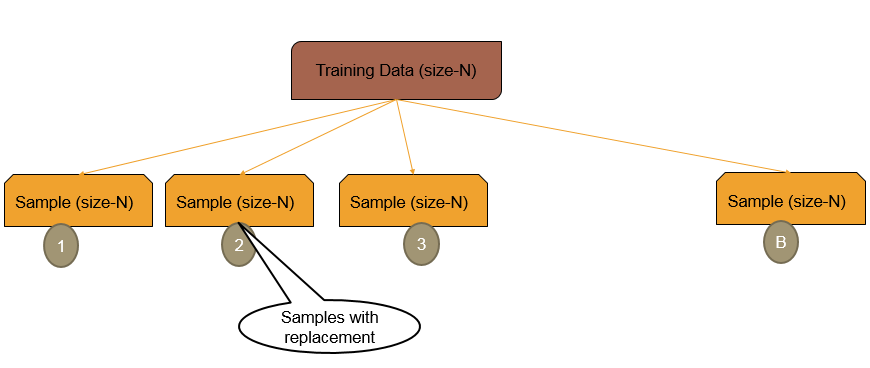### Bootstrap Example

• Example
1. We have a training data is of size 500
2. Boot Strap Data-1:
• Create a dataset of size 500. To create this dataset, draw a random point, note it down, then replace it back. Again draw another sample point. Repeat this process 500 times. This makes a dataset of size 500. Call this as Boot Strap Data-1
1. Multiple Boot Strap datasets
• Repeat the procedure in step -2 multiple times. Say 200 times. Then we have 200 Boot Strap datasets
1. We can build the models on these 200 boost strap datasets and the average error gives a good idea on overall error. We can even use the original training data as the test data for each of the models

### LAB: Bootstrap Cross Validation

• Draw a boot strap sample with sufficient sample size
• Build a tree model and get an estimate on true accuracy of the model

### Solution

• Draw a boot strap sample with sufficient sample size

Where number is B

train_control <- trainControl(method="boot", number=20) 

Tree model on boots straped data

Boot_Strap_model <- train(active_cust~., method="rpart", trControl= train_control, control=rpart.control(minsplit=10, cp=0.000001),  data=Fiberbits)
Boot_Strap_predictions <- predict(Boot_Strap_model)

conf_matrix7<-confusionMatrix(Boot_Strap_predictions,Fiberbits\$active_cust)
conf_matrix7
## Confusion Matrix and Statistics
##
##           Reference
## Prediction     0     1
##          0 28608 11257
##          1 13533 46602
##
##                Accuracy : 0.7521
##                  95% CI : (0.7494, 0.7548)
##     No Information Rate : 0.5786
##     P-Value [Acc > NIR] : < 2.2e-16
##
##                   Kappa : 0.4879
##  Mcnemar's Test P-Value : < 2.2e-16
##
##             Sensitivity : 0.6789
##             Specificity : 0.8054
##          Pos Pred Value : 0.7176
##          Neg Pred Value : 0.7750
##              Prevalence : 0.4214
##          Detection Rate : 0.2861
##    Detection Prevalence : 0.3987
##       Balanced Accuracy : 0.7422
##
##        'Positive' Class : 0
## 

The next post is about Type of Dataset, Type of Errors and Problem of Overfitting.

21st June 2017

### 0 responses on "203.4.4 What is a Best Model?"

Statinfer Software Solutions LLP

Software Technology Parks of India,
NH16, Krishna Nagar, Benz Circle,